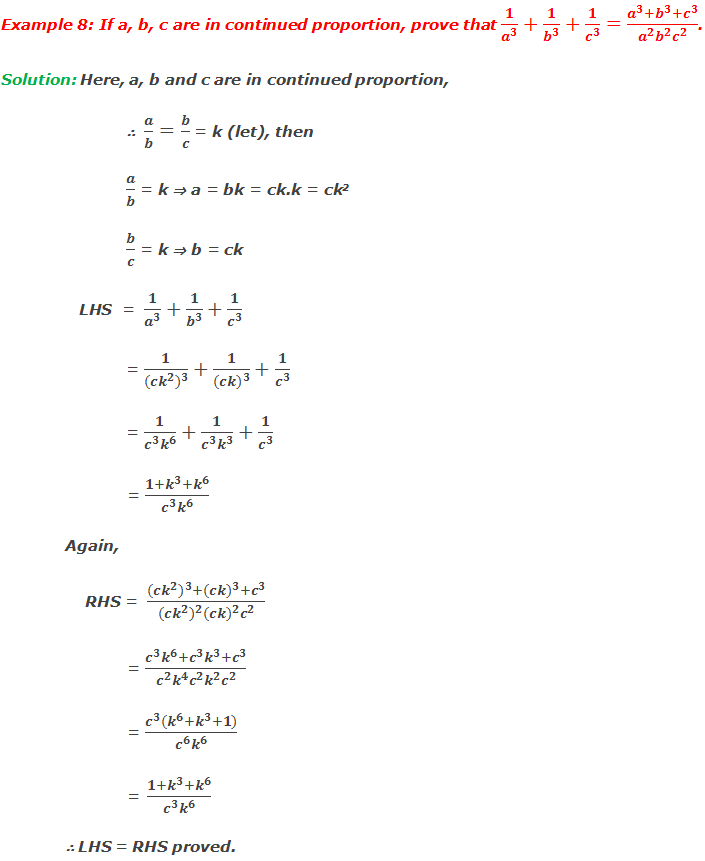# Proportional

## Proportional

If two ratios are equal then they are said to be in proportion or proportional. If a/b = c/d, it is written as a:b::c:d and read as ‘a’ is to ‘b’ is as ‘c’ is to ‘d’. The first term ‘a’ and the fourth term ‘d’ are called extremes and second term ‘b’ and third term ‘c’ are called means.********************

10 Math Problems officially announces the release of Quick Math Solver, an Android App on the Google Play Store for students around the world.

********************

If a:b = b:c = c:d i.e. a/b = b/c = c/d then the terms a, b, c, d are said to be in continued proportion.

If a/b = b/c then b2 = ac. Thus three quantities are said to be in continued proportion if the product of extremes is equal to the square of mean.

### Properties of proportion

If a, b, c and d are four numbers then,

1.   Invertendo:

This property is called as invertendo.

2.   Alternendo:

This property is called as Alternendo.

3.   Componendo:

This property is called as componendo.

4.   Dividendo:

This property is called as dividendo.

5.   Componendo and dividendo:

This property is called componendo and dividendo.

6.   Convertendo or subtendo:

This property is called convertendo or subtendo.

### Some problems on proportional and their solutions:

Example 1: If 5, 10, 15 are in proportion, find the fourth proportion.

Solution: Let x be the fourth proportion. Then 5, 10, 15 and x are in proportion.

Example 2: If 2, x, 6 and 12 are in proportion, find the value of x.

Solution: Here, 2, x, 6 and 12 are in proportion.

Example 3: 4, x and 9 are in continued proportion, find the value of x.

Solution: Here, 4, x, 9 are in continued proportion.

Example 4: What number should be added to each of the numbers 2, 4, 8 and 12 to make them proportional?

Solution: Let the required number be a.